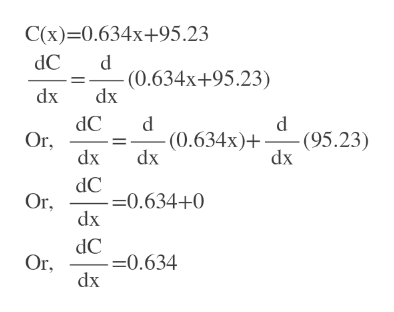# Suppose C(x) measures an economy's personal consumption expenditure and x the personal income, both in billions of dollars. Then the following function measures the economy's savings corresponding to an income of x billion dollars.S(x) = x - C(x)    (Income minus consumption)The quantity dS/dx below is called the marginal propensity to save.dS/dx=1-dC/dxFor the following consumption function, find the marginal propensity to save.C(x) = 0.634x + 95.23

Question
16 views

Suppose C(x) measures an economy's personal consumption expenditure and x the personal income, both in billions of dollars. Then the following function measures the economy's savings corresponding to an income of x billion dollars.

S(x) = x - C(x)    (Income minus consumption)

The quantity dS/dx below is called the marginal propensity to save.

dS/dx=1-dC/dx

For the following consumption function, find the marginal propensity to save.

C(x) = 0.634x + 95.23
check_circle

Step 1

Given marginal propensity is the first derivative of S(x) and given C(x) = 0.634x + 95.23

Step 2

Then we find ...help_outlineImage TranscriptioncloseC(x) 0.634x+95.23 dC d - (0.634x+95.23) dx dx dC Or dx d -(95.23) dx -(0.634x)+ dx dC =0.634+0 dx Or, dC =0.634 dx Or. fullscreen

### Want to see the full answer?

See Solution

#### Want to see this answer and more?

Solutions are written by subject experts who are available 24/7. Questions are typically answered within 1 hour.*

See Solution
*Response times may vary by subject and question.
Tagged in

### Calculus